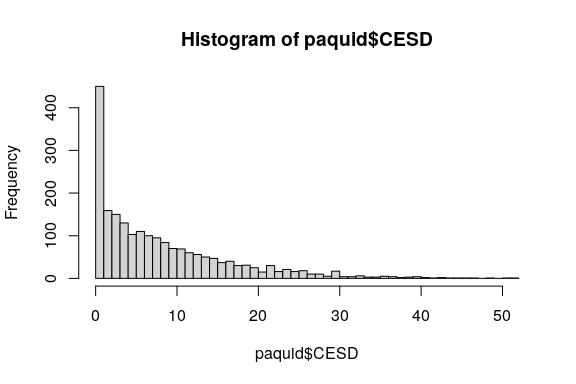# Pre-normalizing a dependent variable using lcmm

Functions lcmm, multlcmm and jointlcmm handle dependent variables that are not necessarily Gaussian. These functions rely on the simultaneous normalization of the variable and estimation of the regression parameters using parameterized link functions (argument “link=”).

However in some cases, one may want to pre-normalize once for all a dependent variable so that standard methods for Gaussian outcomes can then be used without caution.

The methodology has been fully described and validated for MMSE in Philipps et al. (2014) (see https://doi.org/10.1159/000365637 )

We describe here how this can be done using CES-D example.

# CES-D example

CES-D is the scale of depressive symptomatology in the Paquid dataset made of 20 items. Its sumscore is extremely skewed with a large proportion of small values:

summary(paquid$CESD) Min. 1st Qu. Median Mean 3rd Qu. Max. NA's 0.000 2.000 6.000 8.488 12.000 52.000 146  hist(paquid$CESD, breaks=50)# Normalizing a variable with lcmm

The first step is to normalize the variable by estimating a latent process mixed model. This model should roughly fit the data but does not need to be the perfect model or the exact same model as planned for the future complete analysis. One possibility is to define an “empty model” for the covariates (but not the time functions and random effects) :

#We recenter and scale the time variable "age" in order to avoid numerical problems
paquid$age65 <- (paquid$age-65)/10
mpreH <- lcmm(CESD ~ age65 + I(age65^2), random = ~ age65 + I(age65^2), subject = 'ID', data=paquid, link = '5-quant-splines') 

Here a splines link function with 5 knots placed at the quantiles is used.

The variable “obs” of output table “mpreH$pred” includes the normalized values of CES-D for all the observations of the dataset: head(mpreH$pred)
ID     pred_m   resid_m   pred_ss   resid_ss      obs    pred_m1  pred_ss1
1  1 0.11087801 1.3084177 0.9342098  0.4850859 1.419296 0.11087801 0.9342098
2  2 0.06084656 1.2129364 1.4361551 -0.1623721 1.273783 0.06084656 1.4361551
3  2 0.13130626 1.8192130 1.5798633  0.3706559 1.950519 0.13130626 1.5798633
4  2 0.31339434 1.9993094 1.8557171  0.4569866 2.312704 0.31339434 1.8557171
5  2 0.82900547 1.9299116 2.2349518  0.5239653 2.758917 0.82900547 2.2349518
7  3 0.26327450 0.8557631 0.5912821  0.5277555 1.119038 0.26327450 0.5912821

The normalized variable (to be called for instance “normCESD”) can now be added to the dataset

paquid$normCESD <- NULL paquid$normCESD[!is.na(paquid$CESD)] <- mpreH$pred$obs for further analysis. summary(paquid[,c("CESD","normCESD")]) CESD normCESD Min. : 0.000 Min. :-1.8700 1st Qu.: 2.000 1st Qu.:-0.3916 Median : 6.000 Median : 0.5733 Mean : 8.488 Mean : 0.6016 3rd Qu.:12.000 3rd Qu.: 1.5582 Max. :52.000 Max. : 6.1425 NA's :146 NA's :146  # Comparison before and after normalization The transformation does not change the structure of the data. In particular, the spike at 0 is still present. par(mfrow=c(1,2)) hist(paquid$CESD, breaks=50, cex.main=0.9, main="Distribution of CESD")
s <- sd(paquid$normCESD[(paquid$visit==0) & (!is.na(paquid$normCESD))]) paquid$ZnormCESD <- (paquid$normCESD - m)/s ## 2. Rescaling normCESD into 0 - 100 The variable can be scaled in 0-100 with 0 corresponding to the minimum value observed in the sample (usually 0) and 100 the maximum observed value. This works whatever the timescale under study: min <- min(paquid$normCESD[!is.na(paquid$normCESD)]) max <- max(paquid$normCESD[!is.na(paquid$normCESD)]) paquid$normCESD100 <- (paquid$normCESD - min)/(max-min)*100 summary(paquid$normCESD100)
Min. 1st Qu.  Median    Mean 3rd Qu.    Max.    NA's
0.00   18.45   30.49   30.85   42.79  100.00     146 

## Example of model with normCESD100

The statistical analysis can now be performed using one of the normalized variables, normCESD, ZnormCESD or normCESD100.

With normCESD100 for example, a linear mixed model with a linear trajectory according to age with adjustment for male, education and their interaction with time as well as the birth cohort effect (age at entry) can be fitted:

m1 <- hlme(normCESD100 ~ age65*male + CEP*age65 + age_init, random=~age65, subject='ID',data=paquid)
summary(m1)

Or a linear mixed model with a linear trajectory according to time since entry with adjustment for male, education and their interaction with time as well as the birth cohort effect (age at entry):

paquid$time <- paquid$age - paquid\$age_init
m2 <- hlme(normCESD100 ~ time*male + CEP*time + age_init, random=~time, subject='ID', data=paquid)
summary(m2)

Or any other statistical method assuming normality for the outcome!